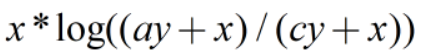# X*log((ay+x)/(cy+x)) a>chow to be expressed by cvx？

Assume

\begin{array}{l} A = ay + x,\\ B = cy + x,\\ \therefore x\log \left( {\frac{{ay + x}}{{cy + x}}} \right) = \left( {\frac{{A - \frac{a}{c}B}}{{1 - \frac{a}{c}}}} \right)\log \left( {\frac{A}{B}} \right) \end{array}
then you have terms like B\log \left( {\frac{A}{B}} \right) and A\log \left( {\frac{A}{B}} \right), those two can be expressed by -rel_entr(B,A) and rel_entr(A,B) respectively, then replace A and B back with the equations above. It might work.

2 Likes

@jackfsuia Very nice. This will be accepted by CVX as a concave expression.

1/(1-a/c)*(rel_entr(a*y+x,c*y+x)+a/c*rel_entr(c*y+x,a*y+x))

This would also work as a convex expression if 0 < a < c.

2 Likes

Very beautiful.that is great. Thank you very much.